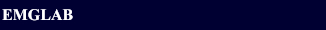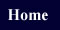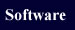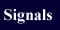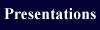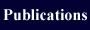EMGLAB FORUMEMGLAB FORUM >Median Frequency

Subject:   Median Frequency

 From:   David Date:   23 Aug 2011 11:24 am Reply via e-mail to David.
I'm trying to find the median frequency of the myopathy signals found in the signal database. Could anyone help with providing matlab code to do this? I am attempting to use the code below where I am guessing and checking for values of f so P=Z, but the results don't seem to be correct.

>> s=emgsignal;
>> s=s.sig;
>> plot(s)
>> Fs=10000;
>> nfft=2*nextpow2(length(s));
>> Pxx=abs(fft(s,nfft)).^2/length(s)/Fs;
>> Hpsd = dspdata.psd(Pxx(1:length(Pxx)/2),'Fs',Fs);
>> plot(Hpsd);
>> p=avgpower(Hpsd)/2
>> Z = avgpower(Hpsd,[0,f])

Thanks